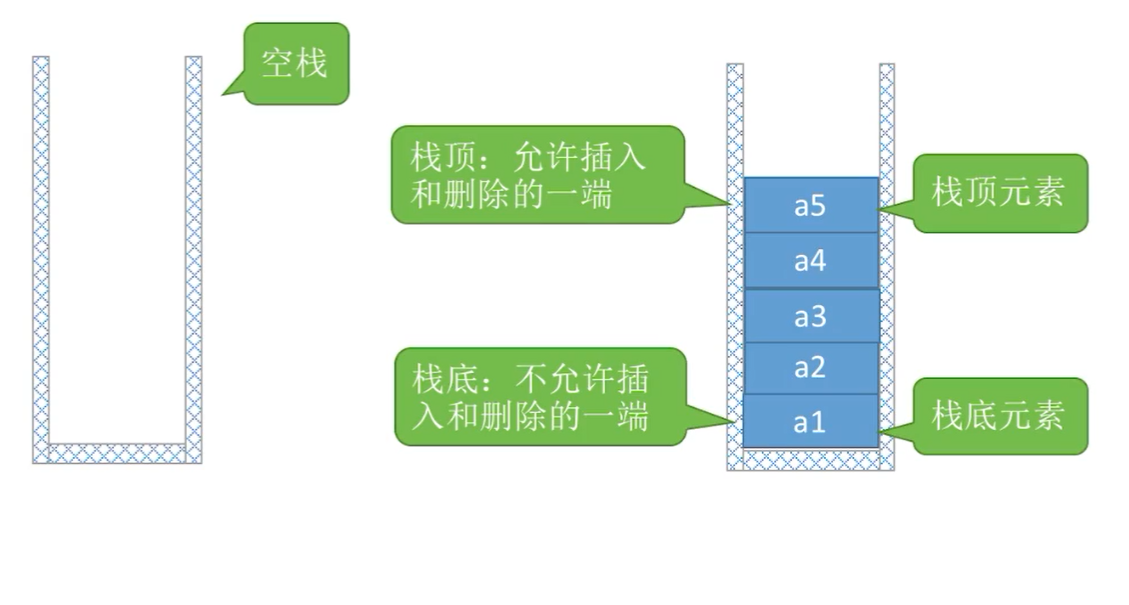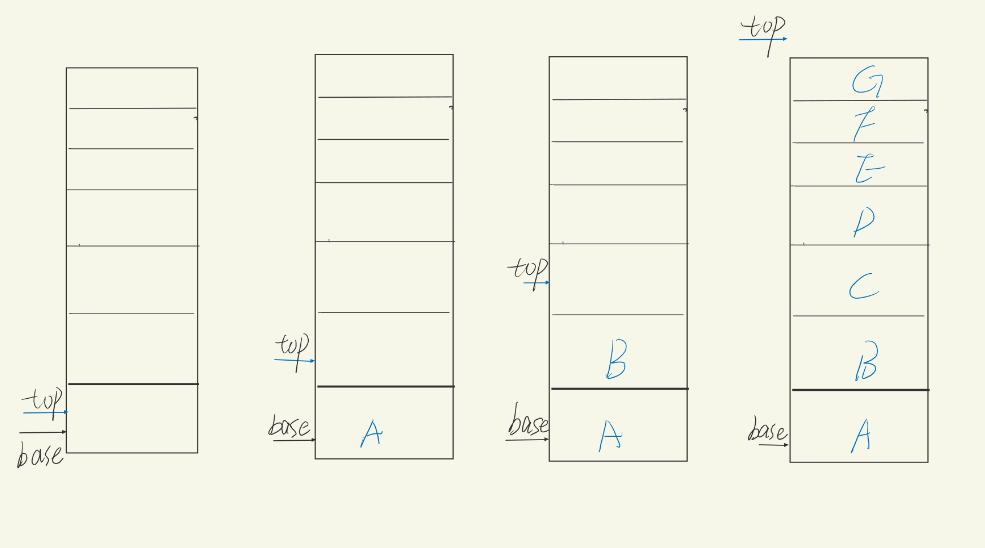## 栈

### 术语• 栈顶 允许插入和删除的一端
• 栈底 ：禁止插入和删除的一端
• 空栈：没有数据元素的栈

### 栈的基本操作

1. InitStack(&S) 初始化一个空栈
2. DestroyStack(&S) 销毁一个栈
3. ClearStack(&S) 清空一个栈
4. Emptystack(S) 判断栈是否为空
5. GetTop(S) 获取栈顶数据
6. Push(&S,e) 将e推入栈中，作为新的栈顶
7. Pop(&S,&e) 推出S的栈顶元素，并用e返回

## 实现---动态顺序栈### 定义

#define InitSize 100 // 初始栈大小

typedef struct {
ElemType* base, * top;// 栈的基址（栈底指针）和 栈顶指针
int stacksize; //栈的大小
}SqStack;


### Init初始化

bool Init_SqStack(SqStack& S)
{
S.base = (ElemType*)malloc(InitSize * sizeof(ElemType));
if (!S.base)return false; //大小开辟失败
S.top = S.base;
S.stacksize = InitSize;
return true;
}


### GetTop 获取栈顶元素

bool GetTop(SqStack S, ElemType& e)
{
if (S.base == NULL)
return false;
e = *(S.top - 1);
return true;
}


### Push元素入栈

bool Push(SqStack& S, ElemType e)
{
if (S.base == NULL)
return false;
if (S.top - S.base >= S.stacksize)//空间不够了，增加空间
{
int* new_base = (ElemType*)realloc(S.top, (S.stacksize + AddSize) * sizeof(ElemType));
if (!new_base) return false;
S.base = new_base;
}
*S.top++ = e;//先赋值后++
return true;
}


### Pop 元素出栈

bool Pop(SqStack& S, ElemType& e)
{
if (S.base == NULL)
return false;
if (S.base == S.top) return false;
e = *--S.top;//先减减再取出元素
return true;
}


### 参考代码

# include<stdio.h>
# include<stdlib.h>

# define InitSize 100

typedef struct {
int* base, * top;// 栈的基址（栈底指针）和 栈顶指针
int stacksize; //栈的大小
}SqStack;

bool Init_SqStack(SqStack& S);
bool GetTop(SqStack S, int& e);
bool Push(SqStack&S, int e);
bool Pop(SqStack&S, int& e);

void test()
{
SqStack S;
int e;
Init_SqStack(S);
printf("入栈数据 1--10\n");
for (int i = 1; i <= 10; i++)
Push(S, i);
printf("出栈5个数据\n\n");
for (int i = 1; i <= 5; i++)
{
Pop(S, e);
printf("此时 数据 ：%3d 出栈\n", e);
}
printf("先进后出\n\n");

getchar();
}

int main()
{
test();
return 0;
}

bool Init_SqStack(SqStack& S)
{
S.base = (int*)malloc(InitSize * sizeof(int));
if (!S.base)return false;
S.top = S.base;
S.stacksize = InitSize;
return true;
}

bool GetTop(SqStack S, int& e)
{
if (S.base == NULL)
return false;
e = *(S.top - 1);
return true;
}

bool Push(SqStack& S, int e)
{
if (S.base == NULL)
return false;
if (S.top - S.base >= S.stacksize)//空间不够了，增加空间
{
int* new_base = (int*)realloc(S.top, (S.stacksize + AddSize) * sizeof(int));
if (!new_base) return false;
S.base = new_base;
}
*S.top++ = e; //先赋值再++
return true;
}

bool Pop(SqStack&S, int& e)
{
if (S.base == NULL)
return false;
if (S.base == S.top) return false;
e = *--S.top; //先-- 再赋值
return true;
}